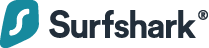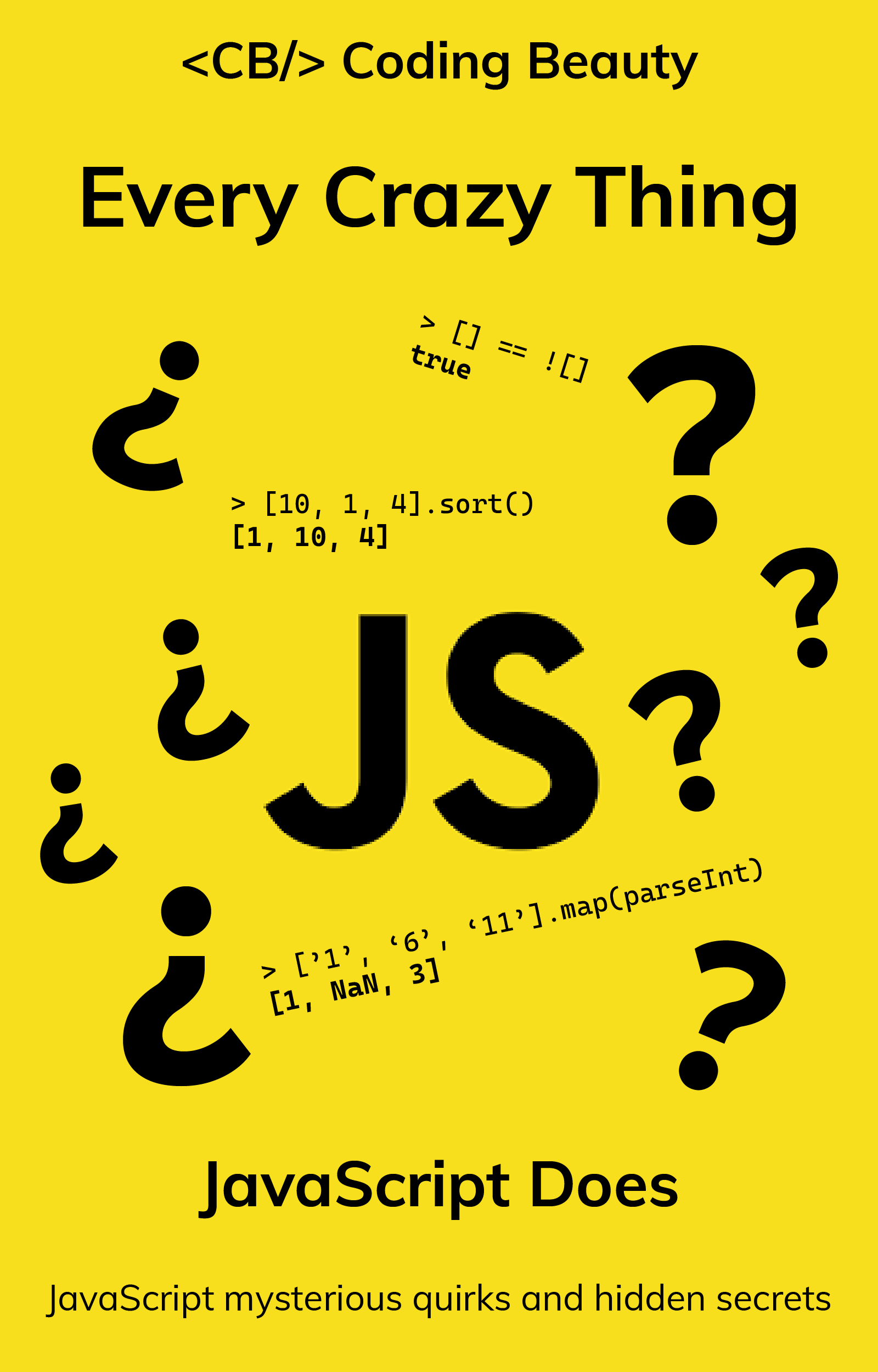# How to Use the JavaScript Nested Ternary Operator

As you might know, ternary operators in JavaScript are a single statement alternative to `if...else` statements frequently used to make code more concise and easier to understand. For example, we could have a function that returns “yes” or “no” depending on whether a number passed to it is odd or not.

JavaScript
``````function isOdd(num) {
if (num % 2 === 1) return 'yes';
else return 'no';
}
``````

We can refactor the `isOdd` function to use a one-line conditional statement like this:

JavaScript
``````function isOdd(num) {
return num % 2 === 1 ? 'yes' : 'no';
}``````

### Surfshark VPN: Your ultimate cyber shieldProtecting your connection is key. Surfing the web, you're exposed to many risks: data breaches, hacking attacks, & snoopers. One solution to fight them all — a VPN.

### Nested ternary operator in JavaScript

We can nest a ternary operator as an expression inside another ternary operator. We can use this to replace if…else if…else statements and switch statements. For example, we could have a piece of code that sets the English word for the numbers 1, 2, and 3 to a variable. With `if...else`:

JavaScript
``````let num = 1;
let word;

if (num === 1) word = 'one';
else if (num === 2) word = 'two';
else if (num === 3) word = 'three';
else num = 'unknown';``````

With `switch...case`:

JavaScript
``````let num = 1;
let word;

switch (num) {
case 1:
word = 'one';
break;
case 2:
word = 'two';
break;
case 3:
word = 'three';
break;
default:
word = 'unknown';
break;
}``````

And now with nested ternary operators:

JavaScript
``````let num = 1;
let word =
num === 1
? 'one'
: num === 2
? 'two'
: num === 3
? 'three'
: 'unknown';``````

The above code example works exactly like the previous two and is less cluttered. Notice we are now able to declare and set the variable in the same statement with this nested ternary approach.

## Every Crazy Thing JavaScript Does

A captivating guide to the subtle caveats and lesser-known parts of JavaScript.